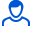Content: 1.zip (440.41 KB)

Positive responses: 0
Negative responses: 0

Refunds: 0Examination number 4.

1. As a result, a sample survey of 100 companies in the region of 500 in the self-repetitions of the random sample the following distribution reducing production costs as a percentage of the previous year.

Reduction

Cost, 4% - 6 6 - 8 8 - 10 10 - December 12 - 14 14 - 16 Total

The number of companies 6 20 31 24 13 6 100

Search:

a) the limits within which the probability 0.907 will be the average percentage of cost reduction on all 500 enterprises;

b) the probability that the proportion of all enterprises, costs which are reduced to not less than 10% different from the proportion of such entities in a sample of not more than 0.04 (in absolute value);

c) the amount of repetitions the sample in which the same boundaries for the average percent reduction of costs (see. p. a) can be guaranteed with a probability of 0.9876.

2. According to the task 1 using - Pearson, at a significance level α = 0,05 to test the hypothesis that the random variable X - the percentage of cost reduction - normal distribution.

Build on one drawing a histogram of the empirical distribution and the corresponding normal curve.

3. Distribution of 60 companies in terms of investment in the development of production X (mln. Rub.) And the resulting profit for the year Y (mln. Rub.) Presented in the table.

y

x 0 - 0.8 0.8 - 1.6 1.6 - 2.4 2.4 - 3.2 3.2 - 4.0 Total

2 - 4 2 April 2

4 - 6 2 7 October 19

6 - 8 February 17th July 26

8 - October 4 3 2 9

10 - 12 February 2

Total 4 11 31 10 4 60

You need:

1. Calculate group averages, build empirical regression line.

2. Assuming that between the variables X and Y there is a linear correlation:

a) Find the equation of the regression lines, build their schedules on a drawing with empirical regression lines and give an economic interpretation of equations;

b) calculate the correlation coefficient; at a significance level α = 0,05 to assess its value and draw a conclusion about the direction of distress and relationships between variables X, and Y;

c) using the appropriate regression equation to estimate the profits in the total investment of 5 million. rubles.

No feedback yet